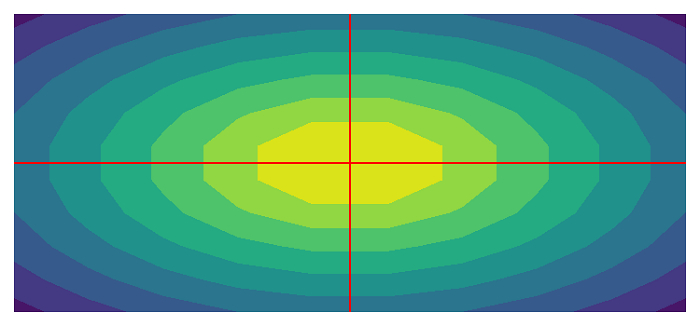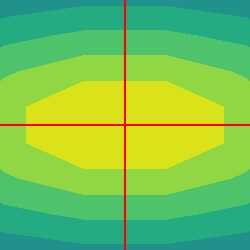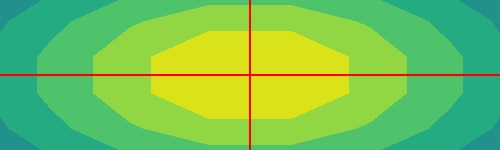# How to crop an image at center in PyTorch?

To crop an image at its center, we apply CenterCrop(). It's one of the transforms provided by the torchvision.transforms module. This module contains many important transformations that can be used to perform manipulation on the image data.

CenterCrop() transformation accepts both PIL and tensor images. A tensor image is a PyTorch tensor with shape [C, H, W], where C is the number of channels, H is the image height and W is the image width.

This transform also accepts a batch of tensor images. A batch of tensor images is a tensor with [B, C, H, W]. B is the number of images in the batch. If the image is neither a PIL image nor a tensor image, then we first convert it to a tensor image and then apply the CenterCrop() transformation.

## Syntax

torchvision.transforms.CenterCrop(size)

### Parameters

• size – Desired crop size. size is a sequence like (h, w), where h and w are the height and width of the cropped image. If size is anint, the cropped image will be a square image.

It returns the cropped image of a given size.

## Steps

We could use the following steps to crop an image at center with a given size.

• Import required libraries. In all the following examples, the required Python libraries are torch, Pillow, and torchvision. Make sure you have already installed them.

import torch
import torchvision
import torchvision.transforms as transforms
from PIL import Image
• Read the input image. The input image is a PIL image or a torch tensor of shape [..., H, W].

img = Image.open('lena.jpg')
• Define a transform to crop the image at its center. The crop size is (200,250) for rectangular crop and 250 for square crop. Change the crop size according your need.

# transform for rectangular crop
transform = transforms.CenterCrop((200,250))

# transform for square crop
transform = transforms.CenterCrop(250)
• Apply the above-defined transform on the input image to crop the image at the center.

img = transform(img)
• Visualize the cropped image

img.show()

## Input Image

The following image is used as the input image in both the examples.## Example 1

The following Python program demonstrates how to crop an image at its center. The cropped image is a square image. In this program, we read the input image as a PIL image.

# Python program to crop an image at center
# import required libraries
import torch
import torchvision.transforms as transforms
from PIL import Image

img = Image.open('waves.png')

# define a transform to crop the image at center
transform = transforms.CenterCrop(250)

# crop the image using above defined transform
img = transform(img)

# visualize the image
img.show()

## Output

It will produce the following output −## Example 2

This Python program crops the image at the center with a given size of height and width. In this program, we read the input image as a PIL image.

# Python program to crop an image at center
# import torch library
import torch
import torchvision.transforms as transforms
from PIL import Image

img = Image.open('waves.png')

# define a transform to crop the image at center
transform = transforms.CenterCrop((150,500))

# crop the image using above defined transform
img = transform(img)

# visualize the image
img.show()

## Output

The resultant output image would be of 150px height and 500px wide.## Example 3

In this program, we read the input image as an OpenCV image. We define a transform which is a composition of three transforms. We first convert the image to a tensor image, then apply CenterCrop(), and finally convert the cropped tensor image to a PIL image.

# import the required libraries
import torch
import torchvision.transforms as transforms
import cv2

# convert image from BGR to RGB
img = cv2.cvtColor(img, cv2.COLOR_BGR2RGB)

# Define a transform. It is a composition
# of three transforms
transform = transforms.Compose([
transforms.ToTensor(),        # Converts to PyTorch Tensor
transforms.CenterCrop(250),   # crops at center
transforms.ToPILImage()       # converts the tensor to PIL image
])
# apply the above transform to crop the image
img = transform(img)

# display the cropped image
img.show()

## Output

It will produce the following output −